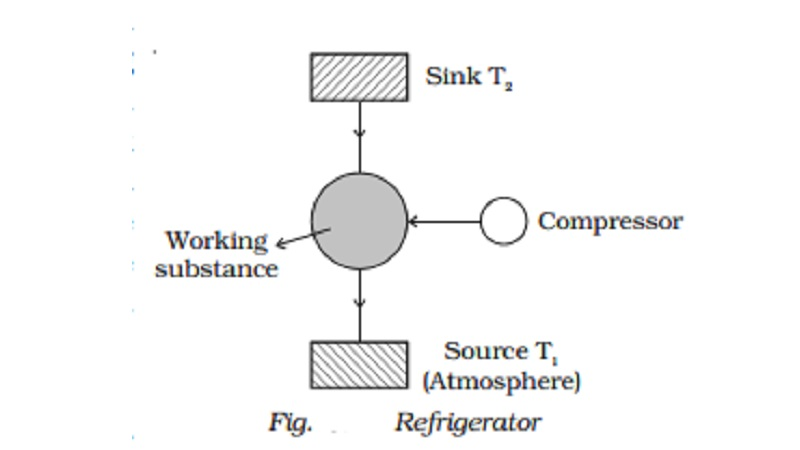Home | | Physics | | Physics | Refrigerator : Coefficient of performance and Inferences

# Refrigerator : Coefficient of performance and InferencesA refrigerator is a cooling device. An ideal refrigerator can be regarded as Carnot?s heat engine working in the reverse direction.

Refrigerator

A refrigerator is a cooling device. An ideal refrigerator can be regarded as Carnot?s heat engine working in the reverse direction. Therefore, it is also called a heat pump. In a refrigerator the working substance would absorb certain quantity of heat from the sink at lower temperature and reject a large amount of heat to the source at a higher temperature with the help of an external agency like an electric motor (Fig.).

In an actual refrigerator

vapours of freon (dichloro difluoro methane CCl2F2) act as the working substance. Things kept inside the refrigerator act as a sink at a lower temperature T2. A certain amount of work W is performed by the compressor (operated by an electric motor) on the working substance. Therefore, it absorbs heat energy Q2 from the sink and rejects Q1 amount of heat energy to the source (atmosphere) at a temperature T1.Since this is a reversible cyclic process, the change in the internal energy of the working substance is zero (i.e) dU = 0

According to the first law of thermodynamics,

dQ = dU + dW

But dQ = Q2 - Q1

dW = -W and dU = 0

dQ=Q2- Q1 = -W

Negative sign with W represents work is done on the system

(i.e) W = Q1 - Q2

Coefficient of performance

Coefficient of performance (COP) is defined as the ratio of quantity   of heat Q2 removed per cycle from the contents of the refrigerator to the energy spent per cycle W to remove this heat.

(i.e) COP = Q2 /W

=Q2 / Q1-Q2

(i.e) COP = T2 / T1-T2     ???..(1)

The efficiency of the heat engine is

η= 1 ?(T2/T1)

1- η = T2/T1

1- η  /  η  =    T2  / T1-T            ????..(2)

From equations (1) and (2)

COP = 1- η  /  η                 ????.(3)

Inferences

(i) Equation (1) shows that smaller the value of (T1 - T2) greater is the value of COP. (i.e.) smaller is the difference in temperature between atmosphere and the things to be cooled, higher is the COP.

(ii)                  As the refrigerator works, T2 goes on decreasing due to the formation of ice. T1 is almost steady. Hence COP decreases. When the refrigerator is defrosted, T2 increases.

Therefore defrosting is essential for better working of the refrigerator.

Study Material, Lecturing Notes, Assignment, Reference, Wiki description explanation, brief detail

Related Topics